# Fraction calculator

The calculator performs basic and advanced operations with fractions, expressions with fractions combined with integers, decimals, and mixed numbers. It also shows detailed step-by-step information about the fraction calculation procedure. Solve problems with two, three, or more fractions and numbers in one expression.

## Result:

### 0.9289 - 0.7493 = 449/2500 = 0.1796

Spelled result in words is four hundred forty-nine over two thousand five hundred.

### How do you solve fractions step by step?

1. Conversion a decimal number to a fraction: 0.9289 = 9289/10000 = 9289/10000

a) Write down the decimal 0.9289 divided by 1: 0.9289 = 0.9289/1
b) Multiply both top and bottom by 10 for every number after the decimal point. (For example, if there are two numbers after the decimal point, then use 100, if there are three then use 1000, etc.)
0.9289/1 = 9.289/10 = 92.89/100 = 928.9/1000 = 9289/10000
Note: 9289/10000 is called a decimal fraction.

c) Simplify and reduce the fraction
9289/10000 = 9289 * 1/10000 * 1 = 9289 * 1/10000 * 1
2. Conversion a decimal number to a fraction: 0.7493 = 7493/10000 = 7493/10000

a) Write down the decimal 0.7493 divided by 1: 0.7493 = 0.7493/1
b) Multiply both top and bottom by 10 for every number after the decimal point. (For example, if there are two numbers after the decimal point, then use 100, if there are three then use 1000, etc.)
0.7493/1 = 7.493/10 = 74.93/100 = 749.3/1000 = 7493/10000
Note: 7493/10000 is called a decimal fraction.

c) Simplify and reduce the fraction
7493/10000 = 7493 * 1/10000 * 1 = 7493 * 1/10000 * 1
3. Subtract: 0.9289 - 0.7493 = 9289/10000 - 7493/10000 = 9289 - 7493/10000 = 1796/10000 = 4 · 449/4 · 2500 = 449/2500
For adding, subtracting, and comparing fractions, it is suitable to adjust both fractions to a common (equal, identical) denominator. The common denominator you can calculate as the least common multiple of both denominators - LCM(10000, 10000) = 10000. In practice, it is enough to find the common denominator (not necessarily the lowest) by multiplying the denominators: 10000 × 10000 = 100000000. In the following intermediate step, cancel by a common factor of 4 gives 449/2500.
In other words - nine thousand two hundred eighty-nine over ten thousand minus seven thousand four hundred ninety-three over ten thousand = four hundred forty-nine over two thousand five hundred.

#### Rules for expressions with fractions:

Fractions - simply use a forward slash between the numerator and denominator, i.e., for five-hundredths, enter 5/100. If you are using mixed numbers, be sure to leave a single space between the whole and fraction part.
The slash separates the numerator (number above a fraction line) and denominator (number below).

Mixed numerals (mixed fractions or mixed numbers) write as integer separated by one space and fraction i.e., 1 2/3 (having the same sign). An example of a negative mixed fraction: -5 1/2.
Because slash is both signs for fraction line and division, we recommended use colon (:) as the operator of division fractions i.e., 1/2 : 3.

Decimals (decimal numbers) enter with a decimal point . and they are automatically converted to fractions - i.e. 1.45.

The colon : and slash / is the symbol of division. Can be used to divide mixed numbers 1 2/3 : 4 3/8 or can be used for write complex fractions i.e. 1/2 : 1/3.
An asterisk * or × is the symbol for multiplication.
Plus + is addition, minus sign - is subtraction and ()[] is mathematical parentheses.
The exponentiation/power symbol is ^ - for example: (7/8-4/5)^2 = (7/8-4/5)2

#### Examples:

subtracting fractions: 2/3 - 1/2
multiplying fractions: 7/8 * 3/9
dividing Fractions: 1/2 : 3/4
exponentiation of fraction: 3/5^3
fractional exponents: 16 ^ 1/2
adding fractions and mixed numbers: 8/5 + 6 2/7
dividing integer and fraction: 5 ÷ 1/2
complex fractions: 5/8 : 2 2/3
decimal to fraction: 0.625
Fraction to Decimal: 1/4
Fraction to Percent: 1/8 %
comparing fractions: 1/4 2/3
multiplying a fraction by a whole number: 6 * 3/4
square root of a fraction: sqrt(1/16)
reducing or simplifying the fraction (simplification) - dividing the numerator and denominator of a fraction by the same non-zero number - equivalent fraction: 4/22
expression with brackets: 1/3 * (1/2 - 3 3/8)
compound fraction: 3/4 of 5/7
fractions multiple: 2/3 of 3/5
divide to find the quotient: 3/5 ÷ 2/3

The calculator follows well-known rules for order of operations. The most common mnemonics for remembering this order of operations are:
PEMDAS - Parentheses, Exponents, Multiplication, Division, Addition, Subtraction.
BEDMAS - Brackets, Exponents, Division, Multiplication, Addition, Subtraction
BODMAS - Brackets, Of or Order, Division, Multiplication, Addition, Subtraction.
GEMDAS - Grouping Symbols - brackets (){}, Exponents, Multiplication, Division, Addition, Subtraction.
Be careful, always do multiplication and division before addition and subtraction. Some operators (+ and -) and (* and /) has the same priority and then must evaluate from left to right.

## Fractions in word problems:

• One Saturday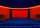One Saturday evening there are 40 girls 25 boys 18 women and 17 men at a cinema. What fraction are girls?
• Lowest terms 2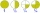The expression 4/12 can be expressed in its lowest term as 1/3. What is 3/15 expressed in its lowest term?
• In the cafeteriaThere are 18 students in Jacob’s homeroom. Six students bring their lunch to school. The rest eat lunch in the cafeteria. In simplest form, what fraction of students eat lunch in the cafeteria?
• SomeoneSomeone ate 1/10 of a cake, leaving only 9/10. If you eat 2/3 of the cake that is left, how much of a whole cake will you have eaten?
• In fractionsAn ant climbs 2/5 of the pole on the first hour and climbs 1/4 of the pole on the next hour. What part of the pole does the ant climb in two hours?
• A class IV.AIn a class there are 15 girls and 30 boys.  What fraction of the class represents the boys?
• Denominator 2Denominator of a fraction is 5 and numerator is 7. Write the fraction .
• A quarterA quarter of the number 72 is:
• Babies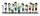There are 2 adults 2 children and 4 babies on a bus. What fraction of the people are babies?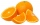If there are 7 apples and 5 oranges in the basket then what fraction of oranges are there in the fruit basket?The correct missing numerator in 2/4 = _/8 to make the fraction equal isWrite as a fraction and a decimal. One and two plus three and five hundredths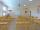In a class of 36 pupils, 2/3 are girls. How many girls and boys are in the class?